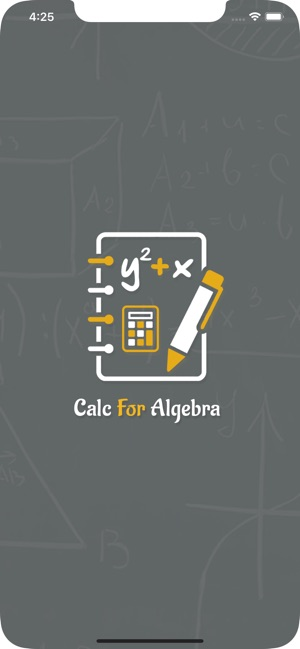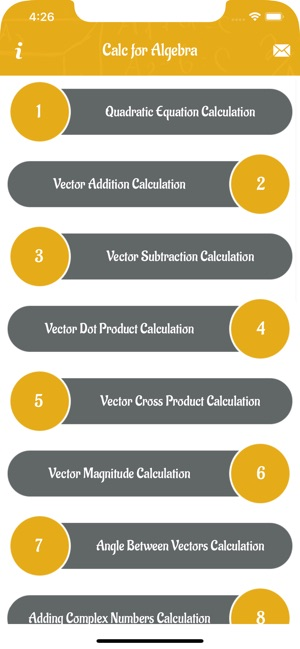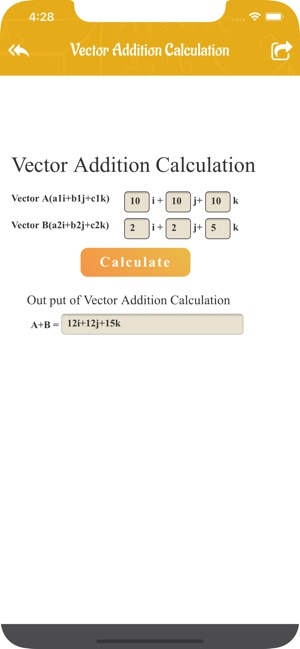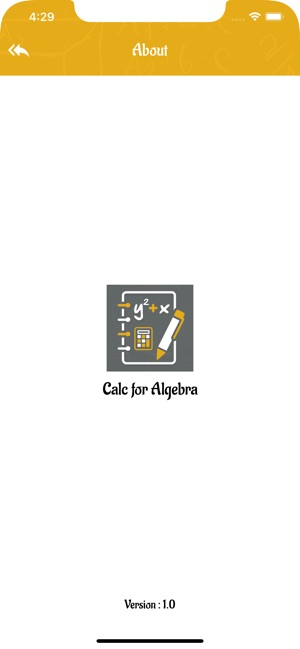## iPhone Screenshots

•••••## Description

Calc For Algebra is a tool to calculate math problem by equations. The main objective of these calculators is to assist students, professionals and researchers quickly perform such calculations easily.

This App Covers:
==============
Vector Subtraction Calculator
Angle Between Vectors Calculator
Complex Numbers Subtraction Calculator
Complex Numbers Multiplication Calculator
Complex Numbers Division Calculator
Polynomial Subtraction Calculator
(a+b)^n Formula Expander
2 Variable Equation Solver
3 Variable Equation Solver
(a-b)^n Formula Expander
Vertex Calculator

## What’s New

Version 1.1

Added push function and fixed bugs.

## Information

Seller
Marian Pearson
Size
26.5 MB
Category
Education
Compatibility

Requires iOS 9.0 or later. Compatible with iPhone, iPad, and iPod touch.

Languages

Simplified Chinese

Age Rating
Rated 4+
•